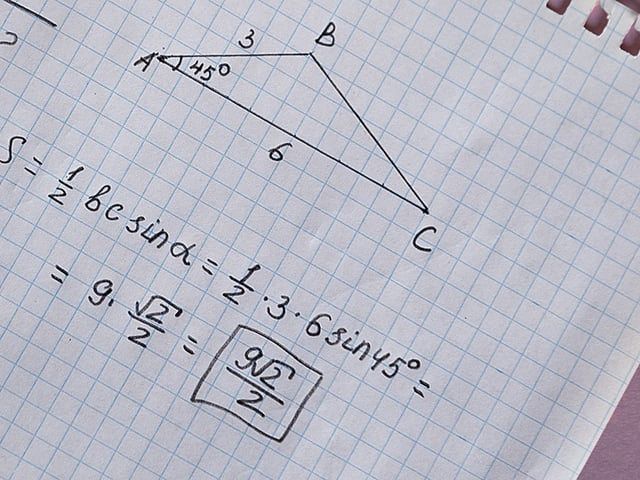# What Does Applied Mathematics Cover on the Workkeys Test?

WorkKeys offers a number of assessments and Applied Mathematics is one of the three required to earn a National Career Readiness Certificate® (NCRS®). The other two are Workplace Documents and Graphic Literacy. The scores on these three tests range from 3 to 7. Based on the assessment scores, the certificate designates one of four levels of achievement: Platinum, Gold, Silver, and Bronze.

## What to Know about the WorkKeys Applied Mathematics Test

The math assessment is timed and you will have 55 minutes to answer 34 multiple-choice questions. This is a web-based examination, and may be taken at a test center. Calculators are permitted, and you will be given a list of formulas which will be needed to solve some of the problems on the test. The test may be taken as many times as is necessary to be successful. It focuses on solving everyday problems using mathematical reasoning and critical thinking.

## What Type of Math Is Assessed on the WorkKeys Applied Mathematics Test?

The test has questions at five levels of difficulty, beginning with Level 3. Each of these require knowledge and skills from the previous level. These are summarized below, and examples are provided for each level:

### Level 3: Solving Simple Problems with One Operation

• Convert fractions to decimals
• Add and subtract positive and negative numbers
• Multiply positive numbers

### Level 4: Using Data Presented Out of Order or in Different Forms

• Solve problems that require more than one mathematical operation
• Calculate the average or mean of a set of numbers
• Utilize simple graphics

### Level 5: Solving Problems That Require Logical Steps and Calculations

• Calculate perimeter, circumference, or area of a shape
• Identify errors in a calculation
• Solve problems with mixed units

### Level 6: Solving Problems That Require Increased Translation and Logic

• Select the proper equation to solve a problem
• Calculate volumes of shapes
• Convert units between measurement systems (e.g., inches to centimeters)

### Level 7: Solving Problems Using Incomplete or Unusual Data

• Use statistical principles to solve problems
• Evaluate alternatives to determine the best value
• Manipulate ratios, rates, and proportions to solve problems

## What Other Types of Problems Are on the WorkKeys Applied Mathematics Test?

Listed below are some of the other areas that may be used as sources of questions:

• Basic addition, subtraction, multiplication, and division
• Totaling bills and calculating change
• Adding multiple monetary amounts and applying sales tax to total bills
• Determining useful information in word problems
• Calculation of production rates
• Finding rates (e.g., miles per gallon)
• Using unit costs to solve problems

## How to Prepare for the WorkKeys Applied Mathematics Test

This test covers a wide range of topics and each question level is more difficult than the previous one. However, many of the questions are based on everyday situations that you have encountered before. The best way to prepare would be by using study guides and taking practice tests. You may know more than you think, and these will help you focus on the areas requiring more practice.

Don’t panic just because you haven’t taken a test in a while. These are everyday problems that we all encounter. Take some time to review what you know and study things you may not remember—and you will be successful. Good luck on the test!